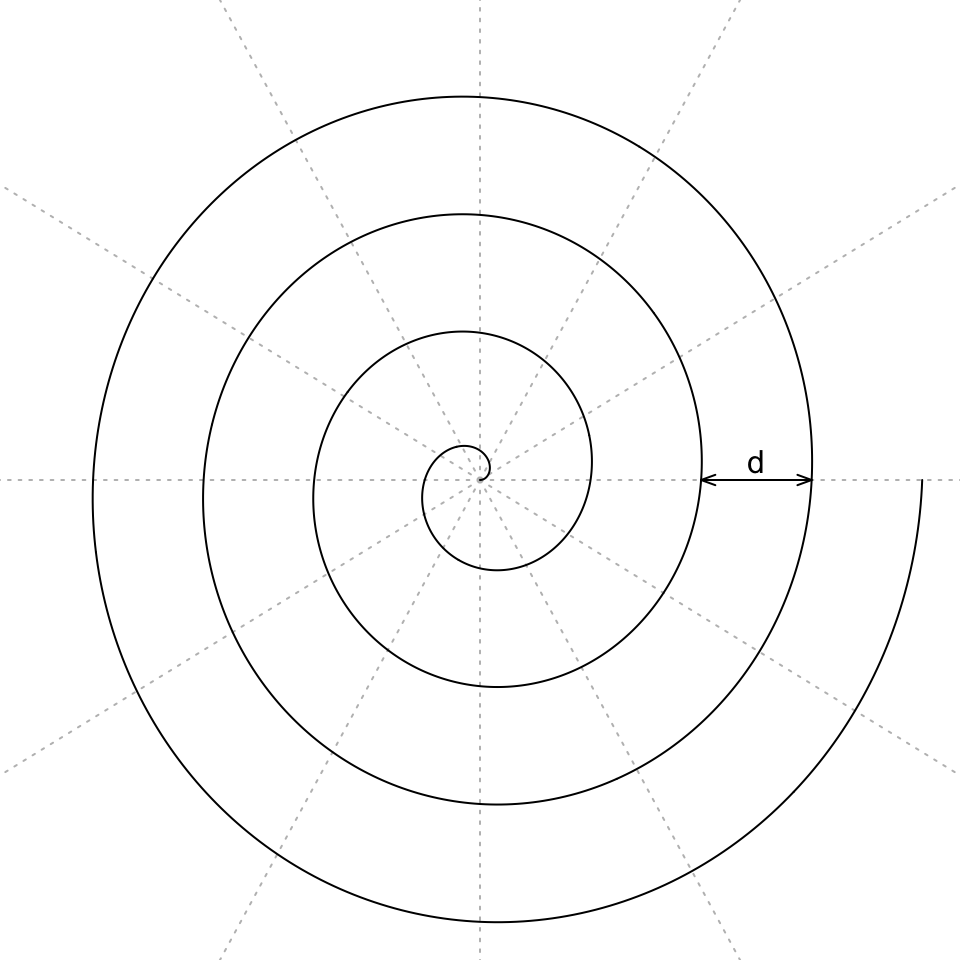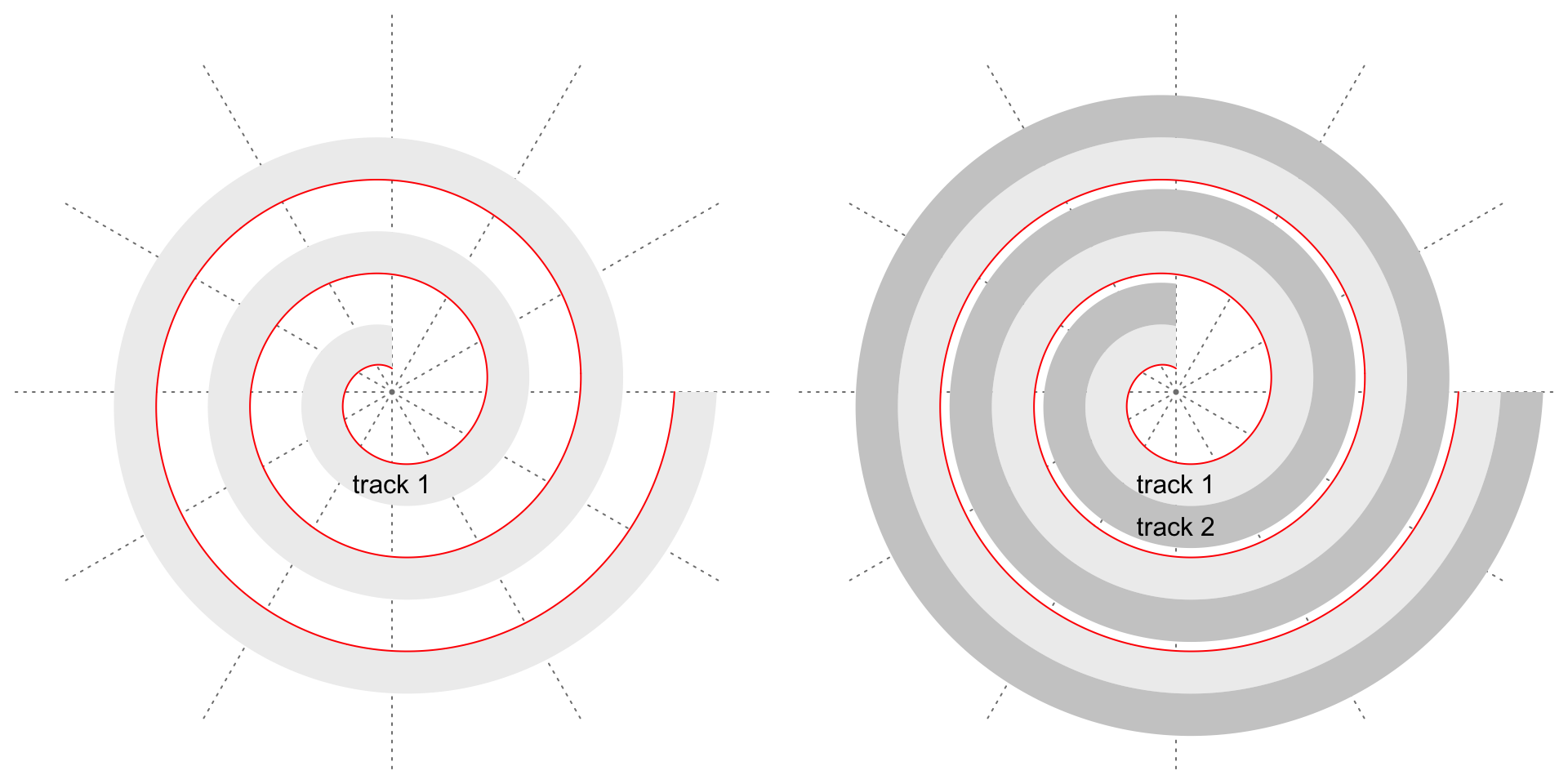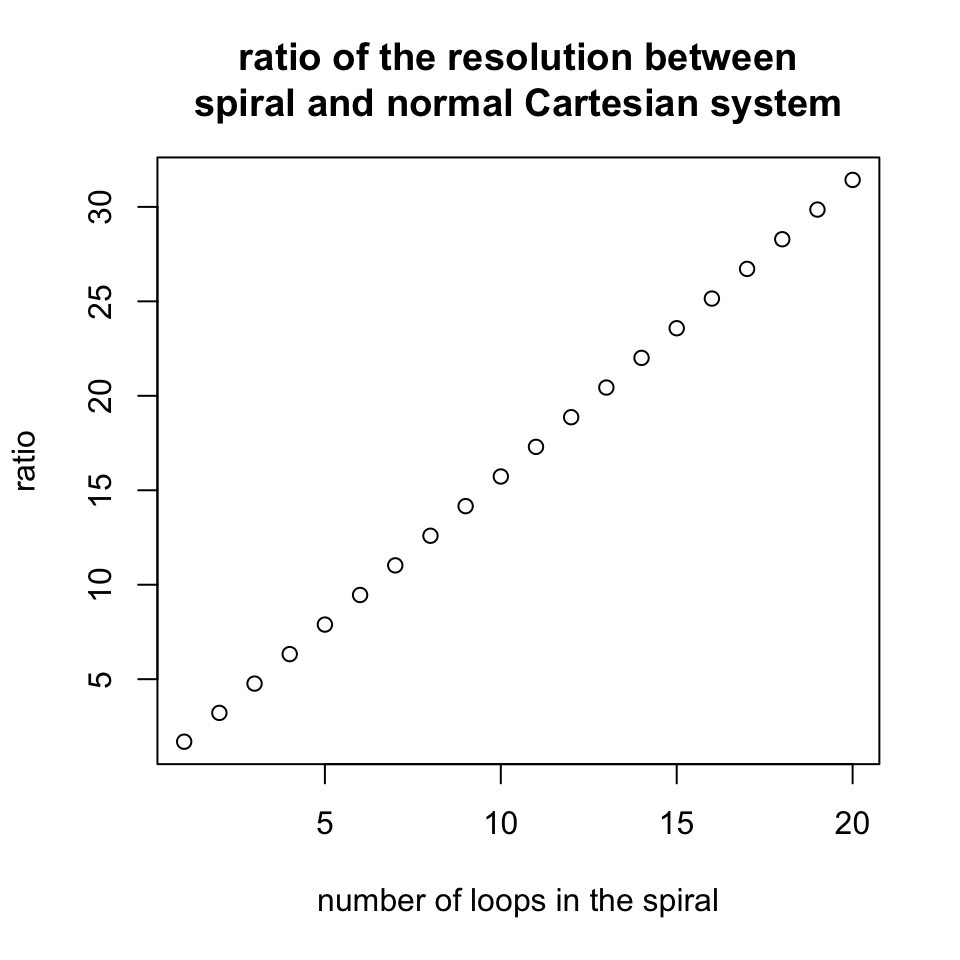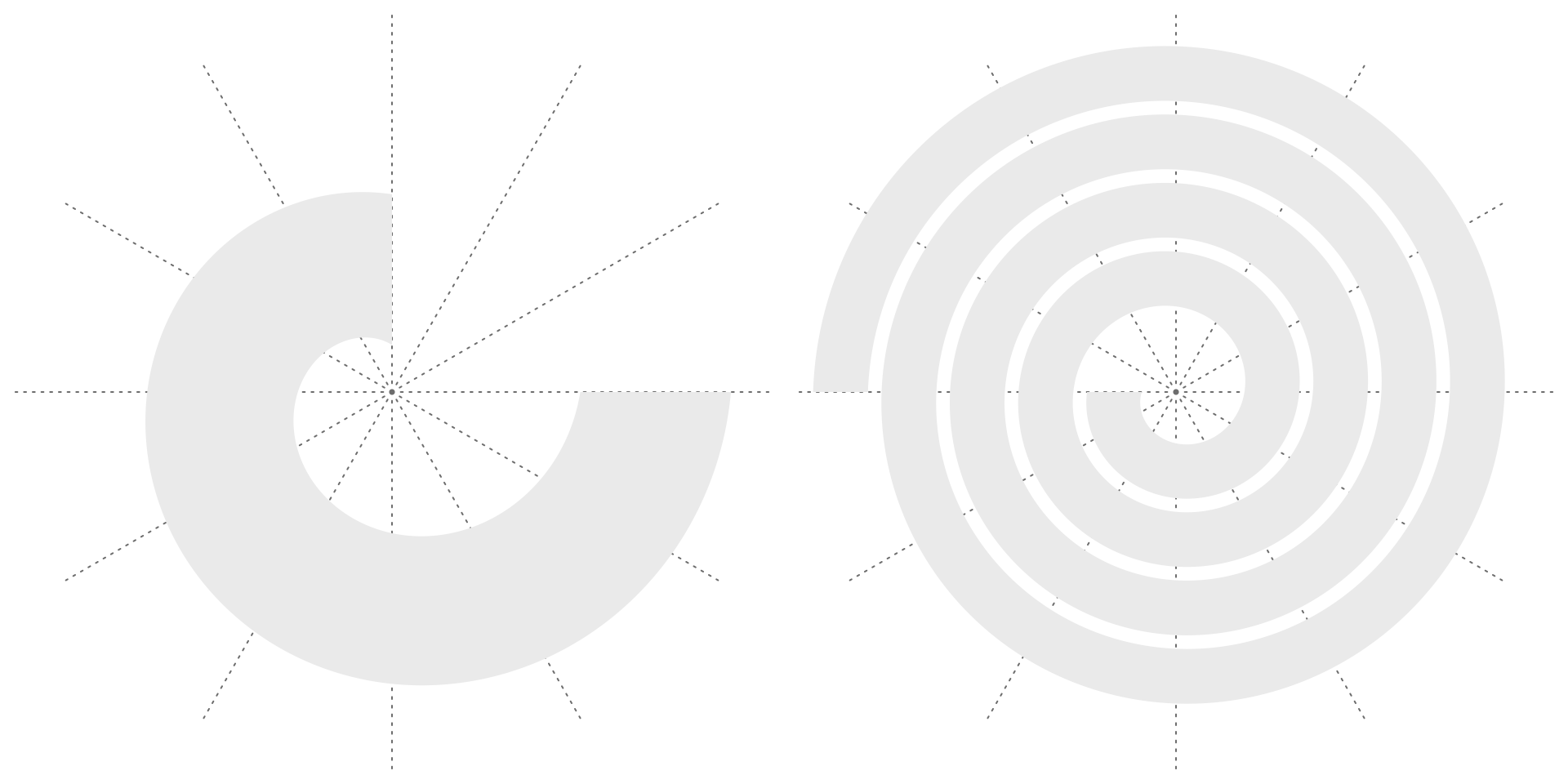# Visualize Data on Spirals

#### 2022-07-26

This vignette is built with spiralize 1.0.5.

In this vignette, I describe the package spiralize which visualizes data along an Archimedean spiral. It has two major advantages for visualization:

1. It is able to visualize data with very long axis with high resolution.
2. It is efficient for time series data to reveal periodic patterns.

## The Archimedean spiral

In polar coordinates ($$r$$, $$\theta$$), the Archimedean spiral has the following form:

$r = b \cdot \theta$

where $$b$$ controls the distance between two loops. The radial distance between two neighbouring loops for a given $$\theta$$ is:

$d(\theta) = r(\theta + 2\pi) - r(\theta) = b \cdot (\theta + 2\pi) - b \cdot \theta = b \cdot 2\pi$

This shows the radial distance between two neighbouring loops is independent to the value of $$\theta$$ and is a constant value. The following figure demonstrates an Archimedean spiral with 4 loops ($$\theta \in [0, 8\pi]$$).Note $$\theta$$ can also be negative values where the spiral is mirrored by y-axis (in Cartesian coordinates). In spiralize, we only consider $$\theta$$ as positive values. The mirrored spiral can be set by the flip argument which is introduced later in this vignette.

Since the distance between any two neighbouring loops for any given $$\theta$$ is constant, it is a ideal place to put tracks along the spiral where the tracks have identical radial heights everywhere. Later the tracks can be served as virtual coordinate systems to map to data. This is why the package is called “spiralize” (to transform a normal Cartesian coordinate system to a curved spiral shape). The following two figures demonstrate a spiral with one track and with two tracks. The red line is the spiral itself. The spiral ranges between $$\pi/2$$ and $$6\pi$$. It is easy to see the upper border of each track is also a spiral but with an offset $$a$$:

$r = a + b \cdot \theta$

where $$a$$ is the offset to the “Base spiral” (the red spiral in the following plots).Denote the maximal radius of the spiral as $$d_{max} = b \cdot \theta_{max}$$, and denote the length of the spiral as $$l$$ (which has a complex form, see https://downloads.imagej.net/fiji/snapshots/arc_length.pdf), we can treat $$2d_{max}$$ as the resolution of the visualization applied in the normal Cartesian coordinate system and $$l$$ as the resolution of the visualization applied on the spiral. Then the ratio of the two resolutions is:

$ratio = \frac{l}{2d_{max}}$

E.g., for a spiral with 5 loops ($$\theta_{max} = 10\pi$$), the ratio is 7.89, which means the spiral improves the resolution of visualization almost to 8 folds. Generally, the ratio increases almost linearly to the number of loops.The relationship between ratio and $$\theta$$ has the following form:

$ratio = \frac{\mathrm{ln}(\theta + \sqrt{1 + \theta^2})}{4\theta} + \frac{\sqrt{1 + \theta^2}}{4}$

When $$\theta$$ gets large,

$ratio \approx \frac{\mathrm{ln}(\theta + \theta)}{4\theta} + \frac{\theta}{4} \approx \frac{\theta}{4}$

Denote $$k$$ as the number of loops, i.e. $$\theta = 2\pi \cdot k$$, then,

$ratio \approx \frac{\theta}{4} = \frac{\pi}{2} \cdot k$

## The layout of the spiral

The function spiral_initialize() is used to intialize the spiral. Arguments start and end control the angular range of the spiral. Here the values should be in degrees and they are converted to radians internally.

In spiralize, the parameter $$b$$ in the spiral equation $$r = b \cdot \theta$$ is set to $$b = 1/2\pi$$, so that the distance between two neighbouring loops is $$d = 1$$. Denote $$\theta_e$$ as the end angle (in radians) of the spiral, the ranges of the viewport (under grid graphics system) on both x-axis and y-axis that draw the spiral are $$[-x, x]$$ where

$x = b \cdot \theta_e + d = 1/2\pi \cdot \theta_e + 1$

The following two plots demonstrate different values of start and end. Also as shown in the following example code, I suggest to set the values of start and end in a form of 360*a + b, e.g. 360*4 + 180, so that it is straighforward to know the positions in the polar coordinates and how many loops there are in the spiral (I think people should feel more natural with degrees than radians).

# the left plot
spiral_initialize(start = 90, end = 360)
spiral_track()

# the right plot
spiral_initialize(start = 180, end = 360*4 + 180)
spiral_track()Argument flip controls how to flip the spiral. It accpets one of the four values: "none"/"horizontal"/"vertical"/"both". Examples are as follows. In the examples, I additionally add the axes in the tracks to show in which direction the data extends along the spiral. I also manually adjust the height of the track to give enough space for axes.

# the top left plot
spiral_initialize(flip = "none") # default
spiral_track(height = 0.6)
spiral_axis()

# the top right plot
spiral_initialize(flip = "horizontal")
spiral_track(height = 0.6)
spiral_axis()

# the bottom left plot
spiral_initialize(flip = "vertical")
spiral_track(height = 0.6)
spiral_axis()

# the bottom right plot
spiral_initialize(flip = "both")
spiral_track(height = 0.6)
spiral_axis()